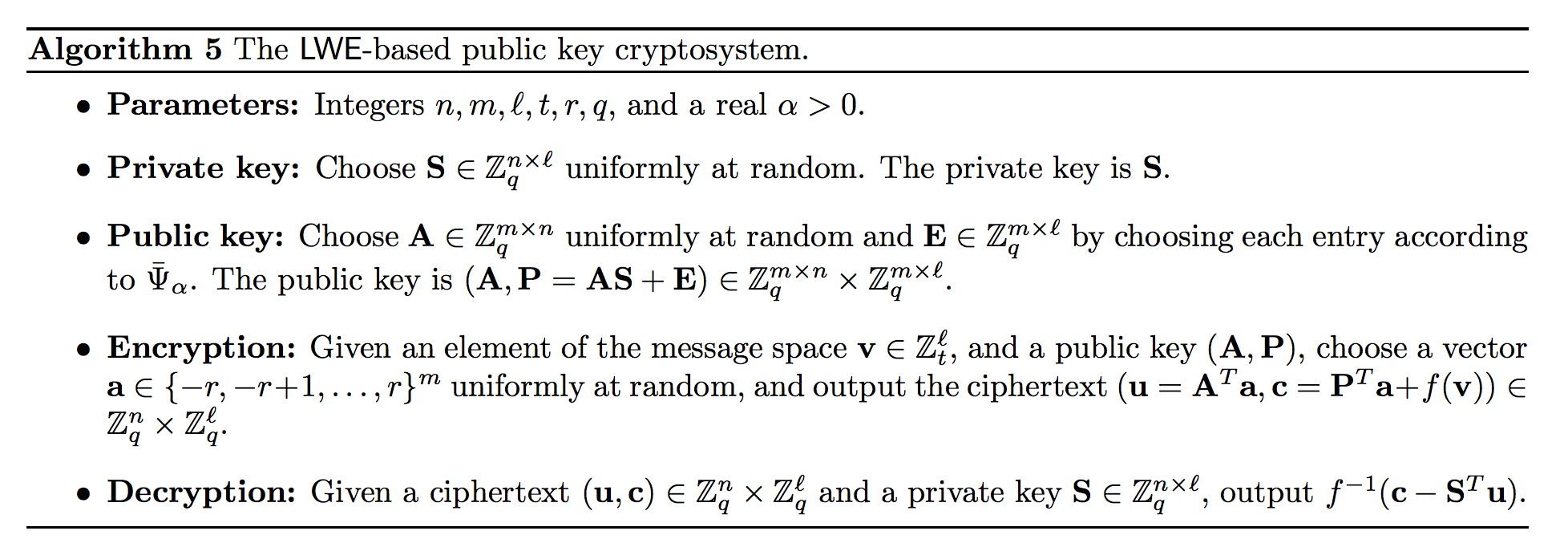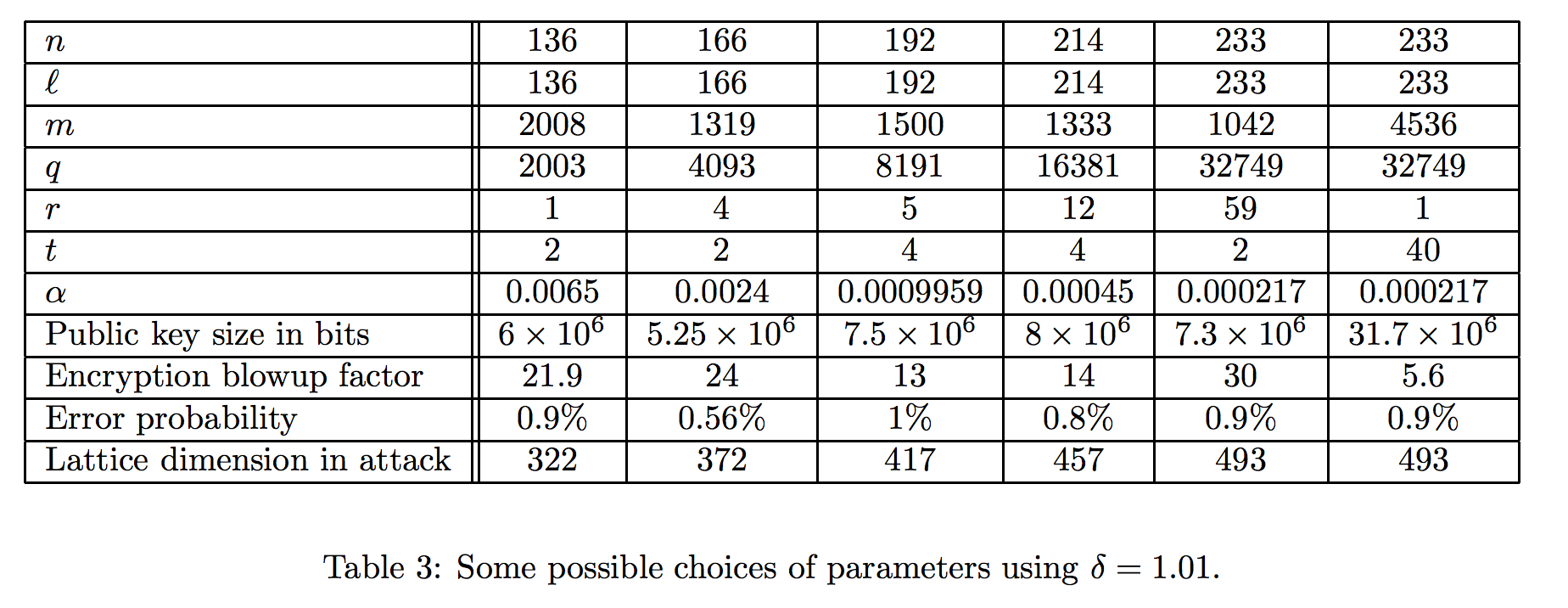# Last Class: The wrap-up

Well, I hope you've picked up tons of crypto skills. More importantly, I hope you've improved your technical resiliency. The ability to take a difficult task that you know little about and push yourself to finish the job is one of the most valuable skills you can possess.

Let's look back at all of the things you've learned so far:

• Brute force analysis
• n-grams
• Frequency Analysis
• Heuristic optimizations
• "Historical" ciphers
• Python skillz
• Hashing
• Salting and Stretching
• Cyclic Groups
• Chinese Remainder Theorem
• Modular Arithmetic
• Finite Fields
• GCD and the Euclidean Algorithm
• Euler's Totient Function
• Computing Inverses
• Repeated squaring
• Pseudo-Random Number Generators
• LFSRs
• LCGs
• Solving wild systems of equations
• Nonces
• Stream Ciphers
• CSPRNGs
• Block Ciphers
• Feistel Networks
• AES
• Various Block Cipher Modes
• Weaknesses in various modes
• Message Authentication Codes
• HMAC
• CBC-MAC
• Secure Channels
• Diffie-Hellman
• El-Gamal
• RSA
• Pohlig-Hellman Attack
• Elliptic Curves
• Quantum Key Exchange
• Homomorphic encryption
• RSA-based digital signatures
• RSA-PSS
• The DSA and ECDSA
• ECDHE
• Threshold CryptoHere are some things we did NOT cover:

• ID-based encryption
• The guts of AES (and DES)
• Blockchains
• Schemes with proofs of difficulty
• Differential Analysis
• Pulling off a side-channel attack
• Realities of setting up PKI

## Last Chance:

I also am very passionate about influencing your lives for the better in the soft skills.

The world needs you to think big about the scope of positive impact you are capable of.

Technology is a powerful tool, and like nuclear energy, can be used for good and for evil. It is your integrity and imagination that is required to move humanity forward.

Here are some influences that I'll passingly drop in your laps hoping they might one-day do you good:

## Last Topic: Group secrets (Threshold Crypto)

OK, so our goal is to cover two types of group-based secrets:

• A message can only be decrypted with the agreement of all participants
• A message can be encrypted with the agreement of any k participants

## A simple 100% group secret

Imagine a secret that requires all of N people to collaborate to unlock the secret.

Then we can do the following:

• m="the message"
• Generate N-1 random strings of the same length as the message there will each be a shared key
• Generate the XOR of the N-1 random strings and m, this will be the last key
• To decrypt just XOR every one of the keys

Group Solve: I made a pretty weak secret hander-outer below. Find a group and push the button until you've got a distinct key. Of course you can just do this yourself too. Find my message!

See the Pen MyRRaX by Andy Novocin (@AndyNovo) on CodePen.

## Threshold Decryption

So now we want to make a secret where each of you gets your own shared key but any k of you can decrypt.

### Shamir's Sharing Scheme

So this one is based on polynomial interpolation which is a great thing to know anyway. The idea is this, imagine that you have to find a polynomial that satisfies $$f(x_1) = y_1$$. There are a lot of answers to that question, but if I tell you that it has to be a polynomial of degree 0 (a constant) then there is just one answer $$f(x)=y_1$$ the horizontal line through that point.

If you give me two points, $$(x_1, y_1), (x_2, y_2)$$ and want to find a polynomial that hits both then there are again many, but only one linear polynomial.

Generally there is a unique polynomial of degree $$t-1$$ that goes through a specified $$t$$ $$(x,y)$$ points.

The scheme:

• Pick a prime modulus, $$p$$, larger than your message, $$m$$, (as an integer) and larger than the number of people you want to have a share of the secret.
• If we need $$t$$ shares to unlock the message then we generate $$t-1$$ random numbers, $$a_1, \ldots, a_{t-1}$$.
• Let your secret polynomial be $$f(x) = m + a_1 \cdot x^1 + \cdots + a_{t-1} \cdot x^{t-1}$$
• Now generate the shares: random $$(x_i, f(x_i))$$ which you give to the stakeholders (everything is modulo $$p$$).
• To decrypt you do polynomial interpolation on $$t$$ shares and that should uniquely produce $$f$$, the message is $$f(0)$$.

I recommend using SAGE to work with polynomials in this case, which requires defining a polynomial ring:

Decrypt using 5 out of 30: I have another weak hander-outer of secrets below. Grab the x and y coordinate of a secret then find a group of 5 people. Find the polynomial that hits those 5 (x,y) coordinates then find f(0) for that polynomial.

See the Pen pyBBwZ by Andy Novocin (@AndyNovo) on CodePen.

If you want to understand how to do polynomial interpolation by hand it goes like this:

For each point $$(x_i, y_i)$$ we want to create a polynomial $$f_i$$ such that $$f_i(x_i) = 1$$ but $$f_i(x_j) = 0$$ for $$x_j \neq x_i$$, then $$\sum f_i(X) \cdot y_i$$ will be a polynomial that satisfies all of our properties (hits each y-value at the right x-value then steps out of the way).

To make that magic polynomial $$f_i$$ we do it like this (the $$i$$ isn't changing but the $$j$$ is):

$$f_i(X) := \frac{\prod_{j=1, j\neq i}^{t}(X-x_j)}{\prod_{j=1, j\neq i}^{t}(x_i - x_j)}$$

For instance if I wanted a polynomial that is 1 at 10 and 0 at 5 and 7 it would be:

$$\frac{(X-5)\cdot (X-7)}{(10-5)\cdot(10-7)}$$

So to interpolate a parabola that hits (10, 2), (5, 1), and (7, 4) off it would look like:

$$\frac{2(x-5)(x-7)}{15} +\frac{(x-10)(x-7)}{10} + \frac{4(x-5)(x-10)}{-6}$$

$$-\frac{13}{30} x^{2} + \frac{67}{10} x - \frac{65}{3}$$

That is called LaGrange Interpolation, and you should meditate on it.

# BONUS TOPIC: LWE public-key scheme (post-quantum crypto)

We have picked up the basic words, ideas, schemes, and google terms to do most of modern industrial crypto.

Now I want to spend a little effort looking into some post-modern crypto. I've picked out an approachable encryption scheme that captures many aspects of this area of research. The area is new (less than a decade of existence) so there are some things that you need to know before you face the bleeding edge:

• These algorithms have "proofs of security" rather than decades of survived attacks
• These algorithms are still in the ivory tower stage because the engineers haven't got to them yet. Never underestimate the power of practitioners.
• The descriptions are still terse because there haven't been enough iterations of books/narratives/explanations. Never underestimate the power of educators.

These papers are harder to read than docs/specs. But if you imagine 3 decades in the future then many classes would put these notions into their syllabi. So the pre-reqs show up in your mind a little faster and you would be different. All of this is to set you up for the idea that, to be cutting edge you've got to find the value in the terse descriptions and not expect someone to chew it for you. If it's easy then you won't get paid.

All that said, I'm going to present this in an "easy" way so you have some framework to explore more on your own.

## The essence of LWE by example

I'm going to present algorithm 5 from this excellent survey paper: https://www.cims.nyu.edu/~regev/papers/pqc.pdf

I feel pretty confident that this idea is what inspired Gentry's development of the first fully homomorphic encryption scheme.OK here goes our take at encrypting one bit (this won't be very secure).

We'll let $$l=n=1$$, $$q=1000000$$, $$t=2$$, $$r=1$$, $$m=3$$.

The big idea is that to encrypt a single message bit which is 0 or 1 we push it to 0 or 500000.

Then we do some linear algebra adjusting and add some small error terms to everything. Now the holder of the secret key can get an almost right answer which rounds to the right value.

Generate Keys: Create (and save) $$A$$ a $$3 \times 1$$ matrix of random values from 0 to 1000000 $$(q)$$. We can use plain arrays for now in this single bit encryption. Generate a secret key $$S$$ as a random number from 1 to 999999. Generate an error vector $$E$$ a $$3 \times 1$$ of numbers picked with normal distribution around 0 and standard deviation $$1000/\sqrt{2\pi}$$. The public key is $$A$$ and $$P= A\cdot S + E$$

To generate normal distribution we can use random.normalvariate(0, 1000./3.)

Publish: put your $$A$$ and $$P$$ out into the chat.

Encrypt: Compute $$r$$ a $$3 \times 1$$ matrix with entries chosen uniformly from -1 to 1. ([random.randint(-1,1) for i in range(3)]) $$U = A^{T} \cdot r$$ (dot product of $$A$$ and $$r$$), and $$C = P \cdot r + mes*500000$$ where $$mes$$ is your secret bit (0 or 1) and everything is mod 1000000.

Publish: Publish $$U$$ and $$C$$.

Decrypt: Decide if $$C - S\cdot U$$ is closer to 500000 or 1000000 mod 1000000.